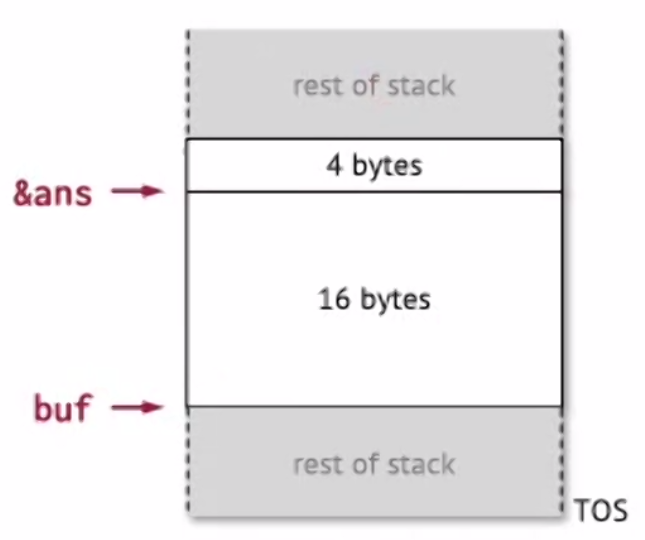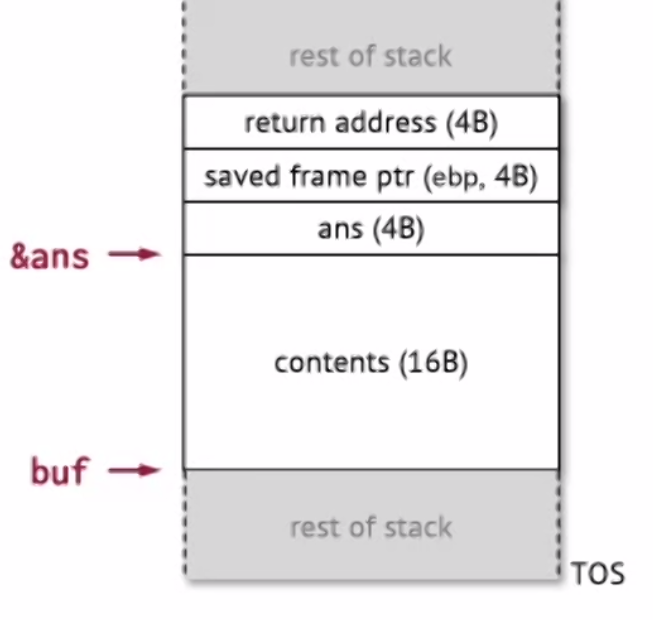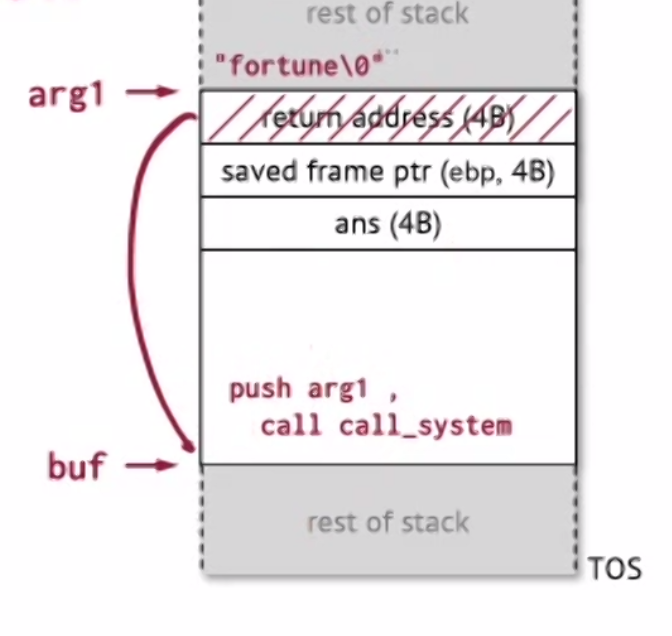# Week 3

Updated 2022-01-25

## Hacks

Recall x86-64 calling conventions:

• We have registers `rax`, `rbx`, `rbx`,…
• When returning, we put the address on the top of stack (TOS)
• We put in arguments in `rdi`, `rsi`, `rdx`,…
• Return values are put in `rax`

In x86 (32-bit) the calling convention is almost the same:

• Have registers `eax`, `ebx`,…
• Return address also goes on TOS
• But all arguments also go on the stack (first argument is right below the return address)
• Return values are put in `eax`

### Buffer Overflow Hack

Knowing this, let’s consider the hack. Let’s make a victim program:

``````int main(int argc, char **argv) {
int ans = 42;
char buf;
gets(buf);
return 0;
}
``````

If we run this code, we get an unsurprising output:

``````the answer is 42
``````

However, there is one vulnerability: the `gets` function reads a line from standard input and puts it into the buffer (char array as arugment) until it finds a terminating character (`\0`) or new line (`\n`). Notice that it does not consider the size of the buffer.

What it means that `gets()` might write past the size allocated (16).

Now let’s come up with our attack to change the answer. To do this we need to reverse-engineer what this code compiles to – to find out where all variables are in memory.In this case, the buffer fills up from bottom to top, so if we input more than 16 bytes we can overwrite the value for `ans`.

So now let’s run the victim program again but we enter the following bytes as standard input:

``````FF FF FF FF FF FF FF FF
FF FF FF FF FF FF FF FF
39 05
``````

Notice that we entered 16-bytes of garbage (who cares) and then two bytes of `0x3905` whch is the lowest two bytes of variable `ans`. When we run the program again the output is

``````the answer is 1337
``````

Knowing this, we can see how this can be used as a typical attack pattern in real system:

• Find a program running as root with file/network inputs
• Scan the binary for insecure functions which might overflow the buffer such as `gets()`, `strcpy()`, etc.
• Then we craft an input to overflow the buffer and to delibrately do whatever we want
• If we can make it execute things like `system("/bin/sh")` then we basically got root-access to the machine. But wait, how do we make it execute something for us?

Lets consider the above victim code, but also consider there are some functions to do some system stuff:

``````// runs a fortune program
int call_fort() { return system("fortune"); }

// calls any system program
int call_sys(const char *s) { return system(s); }
``````

Recall the return address is also on the stack:So if we keep overflowing the buffer, eventually we can overwrite the return address to go to wherever we want.

Suppose we also looked at the binary and found the function `call_fort` to be located at `0x08049765` or `65 97 04 08` in little Endian.

Let’s craft a malicious input byte stream:

``````FF FF FF FF FF FF FF FF <- pads buf
FF FF FF FF FF FF FF FF <- pads buf
FF FF FF FF FF FF FF FF <- overwrites ans and epb
65 97 04 08 <- address of the function for call_fort
``````

The output if we run this is:

``````the answer is: -1
Q: Why did the tachyon cross the road?
A: Because it was on the other side.
 631849 segmentation fault (core dumped)
``````

Note: we successfully used buffer overflow to execute `call_fort` function for us.

Also note: there is segmentation fault because eventually `call_fort` ends and is expecting another return address on the stack but there is none.

Notice earlier we had the `call_sys` function. Ideally we want to pass in whatever argument we want. So how do we do that?

Recall our stack:What we actually can do is to use the buffer to store our arbitraty code, and overwrite the return address to jump to our abitrary code in the buffer, then put the argument somewhere in the stack (we have to put `fortune\0` at the end since in this attack, the insecure `gets` function stops processing when it encounters zero-valued character `\0`):One last thing is that we need to figure out where `buf` is located in memory – again, this can be done by looking at the binary of the program.

Knowing the address of `buf` and the function we want to execute such as `call_sys`, we can use simple arithmetics to compute the addresses.

Note: nowadays stacks are often enforced to be non-executable — effectively breaks our attack we have here.

## Reusing Existing Code

Since we can’t use executable code in the stack, we can exploit existing code that exists in libraries. E.g. ret2lib.

## Return-Oriented Programming

So far we looked at architecture where arguments are stored in the stack to be passed to functions. But a lot of architecture such as ARM, x86-64, etc. has arguments passed via registers.

Instead of injecting new pieces of code, we can find pieces of code we can find in libraries and chaining them together into doing something malicious.

### ROP Hack

To execute a hack, we need to load some value we want into register (e.g. `rdi`). But the problem is that we cannot just simply write to the register.

Luckily we can look for instructions that exists in the program we’re hacking that can write into `rdi`. For example, a `pop` instruction will take something on the stack and put it in some register. Specifically, `pop rdi` will pop something from stack and put it on register `rdi`. Now that we can get some value into the `rdi` register to hack the argument, we also need to jump to some location we want, we can use `ret` instruction.

Ultimately, we want to search the binary for a gadget (series of code) to pop something to `rdi`, then an execute. In x86 binary, we’re looking for specifically the bytes `5f, c3` – which is the gadget encoded in bytes.

On the stack, we can replace the return address with our gadget. The next thing on the stack is the `rdi` value we want to write, and finally, we put another fake-return address (that points to some powerful/root function: e.g. `call_system`) – such that when `ret` executes from the gadget, it will jump to this powerful function.

This hack solves two problems:

1. We don’t need executable stack anymore because we can reuse code from libraries
2. We have a way to “convert” data on the stack to data on the register

### ROP-Chained Hack

If we have multiple arguments we want to hack, then we can chain gadaget address on the stack together. Then the gadget address and the desired argument register values (`rdi`, `rsi`, etc) can all be added to the stack.

A type of defense against stack-abuse by putting a known byte/canary on the stack, and before `ret` is called, the known byte is checked. If it has been changed, then the program will be terminated.